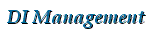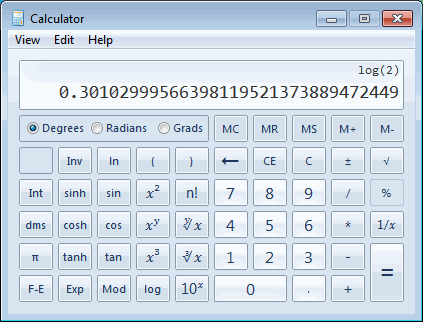DI Management Home > Mathematics > Solving the discrete logarithm problem with bdcalc

#Solving the discrete logarithm problem with bdcalc

This page looks at the discrete logarithm problem and how it can be solved (:-) using bdcalc available from the bdcalc page.

## The logarithm of a real number

The logarithm of a real number $x$ to the base $g$ is defined as the real number $n$ such that $\log_g x=n$ if and only if $x=g^n$.

For example, the logarithm of 2 to the base 10 is approximately 0.30103; that is $\log_{10} 2 \approx 0.30103 \quad \iff \quad 10^{0.30103} \approx 2.0.$

You can easily compute these logarithms on your calculator or (for older readers) use your book of log tables.To compute a logarithm to an arbitrary base $b$ given logarithms to a known base $a$, you can use the relation $\log_b x = \dfrac{\log_a x}{\log_a b}$. For example, if we can only compute $\ln x = \log_e x$ then $\log_{10} 2 = \dfrac{\log_e 2}{\log_e 10} = \dfrac{0.69315}{2.30259}= 0.30103$.

## Discrete logarithm modulo p

The discrete logarithm is similar to the logarithm of a real number but for integers modulo a prime $p$. The discrete logarithm of an integer $x$ to the base $g$ modulo $p$ is defined as the integer $n$ such that $x = g^n \pmod{p}$. That is $\log_g x \equiv n \pmod{p} \quad \iff \quad x \equiv g^n\pmod{p}.$

For example,
• The discrete logarithm of 1 to the base 2 mod 5 is 4 since $2^4 \equiv 1 \pmod{5}$.
• The discrete logarithm of 18 to the base 5 mod 23 is 12 since $5^{12} \equiv 18 \pmod{23}$.
• The discrete logarithm of 3668993056 to the base 5 mod 9048610007 is 948603 since $5^{948603} \equiv 3668993056 \pmod{9048610007}$.

## The discrete logarithm problem

The discrete logarithm problem for the multiplicative group $\mathbb{Z}_p^*$ can be stated as:

Given $g, x\in \{1,2,\ldots,p-1\}$ find $n$ such that $x\equiv g^n\pmod{p}$.

This turns out to be a difficult problem to solve, and gets harder as $p$ gets larger. There is no known efficient algorithm to solve this problem and it is intractable for large $p$ of the order of, say, $2^{1024}$.

## Using bdcalc to solve the discrete logarithm problem

OK, we're joking now. But you can do a simple trial multiplication to solve the problem for reasonably small values of $p$.

The algorithm is as follows. Set $b=1$ and for each $k=1,2,3,\ldots,p-1$ compute $b=bg\pmod{p}$ until $b=x$ then stop and return the value of the counter $k$. This is the discrete logarithm value we require.

We can do this using bdcalc v2 as follows:

> x=1;g=2;p=5  # INPUT
> b=1;for k in (1..p) do b=modmul(b,g,p);breakif(b==x) done;
println("The discrete log of ",x," to the base ",g," mod ",p," is ", k)
The discrete log of 1 to the base 2 mod 5 is 4

> x=18;g=5;p=23  # INPUT
> b=1;for k in (1..p) do b=modmul(b,g,p);breakif(b==x) done;
println("The discrete log of ",x," to the base ",g," mod ",p," is ", k)
The discrete log of 18 to the base 5 mod 23 is 12

> x=3668993056;g=5;p=9048610007  # INPUT
> b=1;for k in (1..p) do b=modmul(b,g,p);breakif(b==x) done;
println("The discrete log of ",x," to the base ",g," mod ",p," is ", k)
The discrete log of 3668993056 to the base 5 mod 9048610007 is 948603

We can verify our results using the modexp() function, showing that modexp(g,k,p) == x as follows
> modexp(2,4,5)
1
> modexp(5,12,23)
18
> modexp(5,948603,9048610007)
3668993056
>


A version of the above code in a script.

The bdcalc calculator for large natural numbers is available from our bdcalc page.